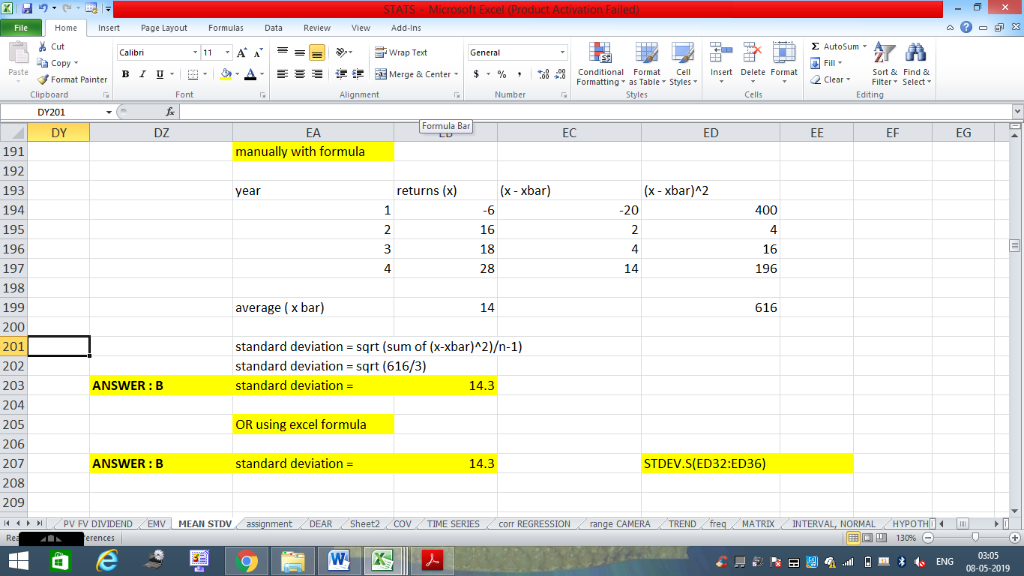In: Finance

# Sun Corporation has had returns of -6 percent, 16 percent, 18 percent, and 28 percent for...

Sun Corporation has had returns of -6 percent, 16 percent, 18 percent, and 28 percent for the past four years. Calculate the standard deviation of the returns using the correction for the loss of a degree of freedom.

 A 11.6 percent B 14.3 percent C 13.4 percent D 14.0 percent E

## Solutions

##### Expert Solution

I HAVE SOLVING USING FORMULA AND ALSO EXCEL FORMULA## Related Solutions

##### A stock had returns of 10 percent, -4 percent, 4 percent, and 18 percent over the...
A stock had returns of 10 percent, -4 percent, 4 percent, and 18 percent over the past four years. What is the standard deviation of these returns?
##### A stock had returns of 5 percent, 9 percent, 11 percent, −8 percent, and 6 percent...
A stock had returns of 5 percent, 9 percent, 11 percent, −8 percent, and 6 percent over the past five years. What is the standard deviation of these returns? Multiple Choice 11.14 percent 7.44 percent 8.44 percent 4.60 percent 5.97 percent
##### A stock has returns of −6 percent, 12 percent, 5 percent, 10 percent, and −9 percent....
A stock has returns of −6 percent, 12 percent, 5 percent, 10 percent, and −9 percent. What are the arithmetic and geometric returns? (Do not round intermediate calculations. Enter your answers as a percent rounded to 2 decimal places.) Arithmetic return% ? Geometric return% ?
##### a stock has had returns of 15 percent, 26 percent, -20 percent and 5 percent over...
a stock has had returns of 15 percent, 26 percent, -20 percent and 5 percent over the last 4 years. what is the holding period return for the stock?
##### A stock has had returns of -26 percent, 12 percent, 34 percent, -8 percent, 27 percent,...
A stock has had returns of -26 percent, 12 percent, 34 percent, -8 percent, 27 percent, and 23 percent over the last six years. What are the arithmetic and geometric average returns for the stock? (Do not round intermediate calculations and enter your answers as a percent rounded to 2 decimal places, e.g., 32.16.) 10.33% Arithmetic average return Geometric average return
##### A stock has had returns of −30 percent, 20 percent, 34 percent, −20 percent, 37 percent,...
A stock has had returns of −30 percent, 20 percent, 34 percent, −20 percent, 37 percent, and 23 percent over the last six years. a.) What are the arithmetic average returns for the stock? b.) What are the geometric average returns for the stock? (4 Points)
##### Over the past four years, a stock produced returns of 13, 6, -5, and 18 percent,...
Over the past four years, a stock produced returns of 13, 6, -5, and 18 percent, respectively. What is the standard deviation of these returns? What would be the standard deviation of these returns if they were exactly twice the return shown for each year? please show your work.
##### A stock has had returns of 10 percent, 8 percent, -25 percent over the last three...
A stock has had returns of 10 percent, 8 percent, -25 percent over the last three years, respectively. What is the geometric mean return for this stock?(Enter your answers as a percentage rounded to 2 decimal places. For example, enter 8.43% instead of 0.0843 ) Answer: Units:
##### returns of 6 percent, 14 percent, 13 percent, 9 percent, and -4 percent for the past...
returns of 6 percent, 14 percent, 13 percent, 9 percent, and -4 percent for the past five years, respectively. What is the standard deviation of these returns
##### REIT B (REIT is a real estate investment trust) stock had returns of 12 percent, 6...
REIT B (REIT is a real estate investment trust) stock had returns of 12 percent, 6 percent, 14 percent, and -3 percent annually for the past four years. What is the mean and standard deviation of these returns? A. 7.25%; 13.22% B. 7.25%; 7.63% C. 8.75%; 9.11% D. 7.25%; 11.08% E. 8.75%; 10.29%